# Vector GAN

Training GAN to generate vector images.

### TLDR

We trained and evaluated several GAN models in a field of vector image generation. The model outputs parameters for a fixed set of drawing primitives (curves and circles), contrary to common “raster” GAN, which generate fixed size image. Despite being trained with 64x64 px image dataset, such model can generate sketches at any resolution, due to using vector graphics.

To achieve this we use differentiable rasterizer from and train different architectures with various datasets.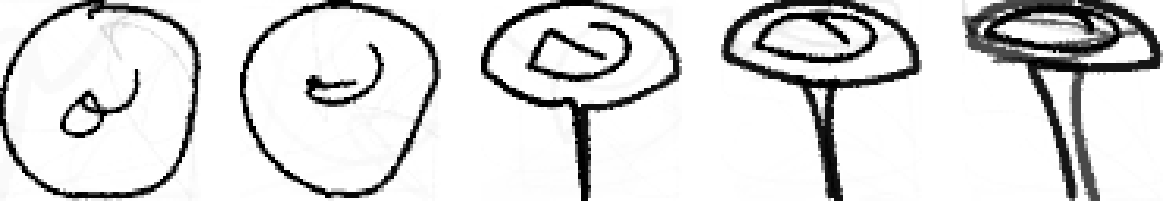Typical GAN generates raster image of a fixed resolution.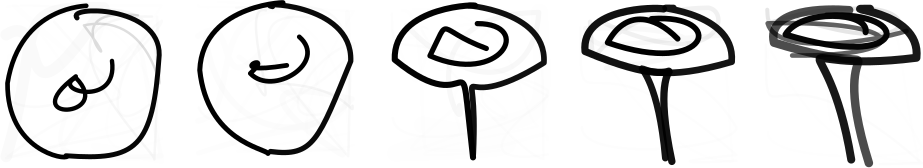Our GAN generates vector graphics, which can be viewed at any resolution and edited easily without loss of quality.

Disclaimer: This is a project I did for IFT 6756: Game Theory and ML

### Motivation

Vector graphics allows for editing without loss of quality. It stores images as a collection of primitives (lines, bezier curves, circles, etc.) with corresponding control parameters (color, width, control points).

Typical generative models are trained on raster images (image as array), while vector graphics space is non-euclidean and thus more challenging. Several approaches were tried, until finally a differentiable rasterizer proposed in made it possible to do a direct backpropagation to primitives parameters.

In this project we apply differentiable rasterizer from to experiment with generative art on different datasets, GAN architectures, and create adversarial examples using painting. We show that it may become a nice tool in media art creation, as it connects capable GANs and other neural nets with all the advantages of vector graphics.

Transparency: the referenced paper already provides the examples of GAN model trained using their rasterizer. The aim of this project was to reproduce their result independently, try another application and new data.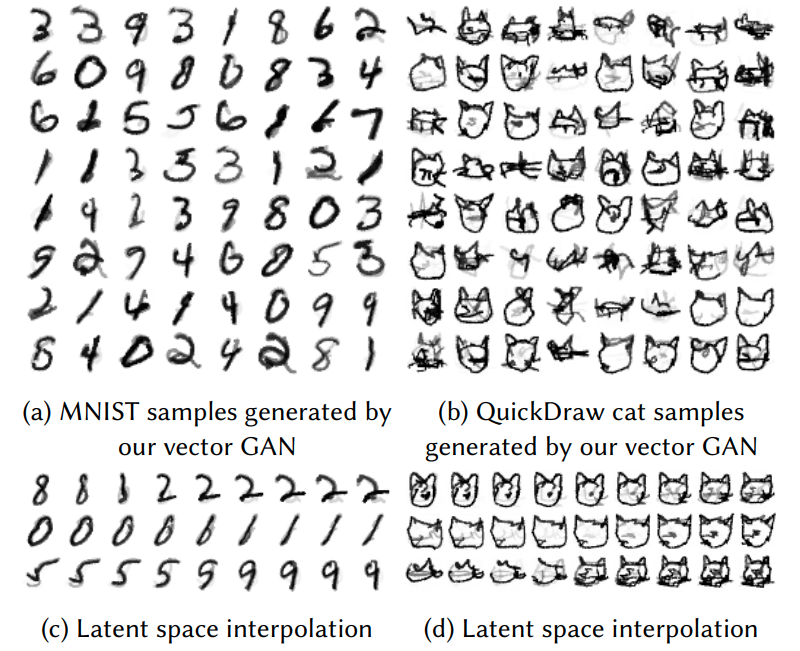Most generative adversarial networks are generating raster images. DoodlerGAN aimed to replicate human drawings by generating raster body parts.

SketchRNN modeled drawing as a decision making sequential process and trained seq2seq RNN VAE to generate quick sketches. SVG VAE applies the same approach to model fonts as a sequence of 4 commands, SketchBERT moved to a next level by training a giant BERT model on the same data. DeepSVG uses transformers to generate SVG control commands for a vider family of svg primitives.

Differentiable rasterizer proposed in allows gradient flow from rendered image to svg parameteres, thus we can optimize svg primitives directly from raster signal. They also showed several application of generative models. Im2Vec uses this rasterizer to generate vector graphics from raster examples.

## Experiments

#### Dataset

We took simple sketches from QuickDraw dataset with categories apple, cookie, donut, face, lollipop and 5000 of each category with resolution 64x64. Note that we train a “grayscale” model to save computations and fit to grayscale dataset, while it is possible to have colored sketches.Dataset examples from QuickDraw data.

We also tried training on more complex classes like owl or another dataset Creative Birds, but due to computational limitations our model did not converge to any good looking results.

#### GAN architectures

Generator takes as input a latent vector $z \in \mathbb{R}^{100}$ and outputs parameters for 16 bezier curves (14 positional parameters + width and alpha) and 5 circles (2 positional parameters + radius + width + alpha). Neural net consists of 4 flat linear layers of dimensions [128, 256, 512, 1024], the last layer is then projected into each parameter family independently. Output parameters is then grouped and passed to differentiable rasterizer to generate image.

Discriminator is a convolutional neural network of 6 layers, with LeakyReLU activation. After experiments we ended up using the same discriminator architecture as was proposed in the original paper, as our attempts failed due to discriminator net being too powerful.

We implemented and trained the following modifications for training GAN, commonly used in literature.

Training of each model took approximately 10 hours on a single GPU (NVidia RTX2080Ti). Unfortunately, we could not use Google Colab as differentiable rasterizer was not compatible with Colab hardware. Number of experiments was also limited due to high computational costs.

• WGAN makes a critic $K$-Lipschitz by clipping its gradients to stabilize training
$\omega \leftarrow clip (\omega, -0.01, 0.01)$
• WGAN-GP forces $K$-Lipschitz by adding a gradient penalty to the loss
$\hat x \leftarrow \epsilon x_{real} + (1-\epsilon) x_{fake}$ $loss \leftarrow D(x_{fake}) - D(x_{real}) + \lambda (\| \nabla_{\hat x} D(\hat x) \|_2 - 1)^2$
• SNGAN Force 1-Lipschitz continuity by spectral normalization of critic’s layers
$\bar W_{SN} (W) = \frac W {\sigma(W)}$
• LOGAN More gradient flow to generator by making a latent gradient step $z = z + \Delta z$ before passing image to discriminator
$g = \frac{\partial D(G(z))}{\partial z}; \; \; \Delta z = \frac {\alpha} {\beta + \| g \|^2} \cdot g$

Unfortunately, LOGAN model did not converge in our experiments - it must be a bug in our code, or a conceptual mistake.

### Evaluation

We evaluated and compared our models using Inception Score IS and Frechet Inception distance FID. Inception Score uses pretrained classificator and measures the variety of generated examples through measuring distribution of labels predicted by pretrained classifier. Frechet inception distance measures this variety by comparing feature distribution extracted by classifier on validation set and generated samples:

$\| \mu_{real} - \mu_{fake} \|_2^2 + Trace(\Sigma_{real} + \Sigma_{fake} - 2 (\Sigma_1 \Sigma_2)^{0.5})$

FID score between validation and test subsets is 30.56

### Results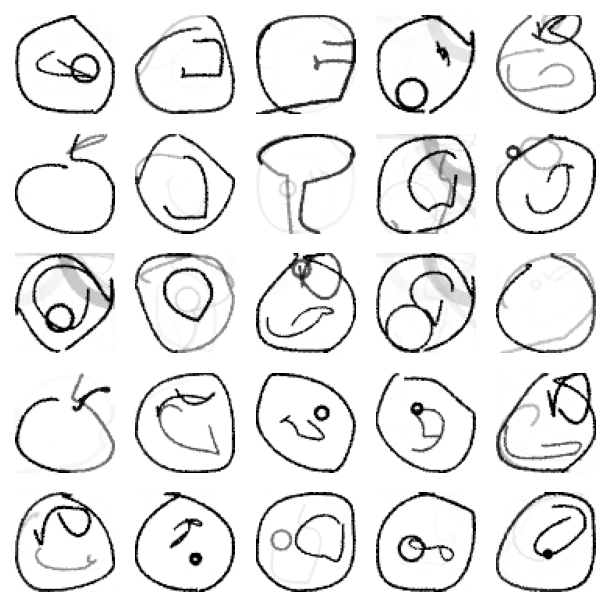Generated data samples. Note that both vector curves and circles are in use here. Images are rasterized to 64x64 resolution for visualization purposes.

As for quality scores, neither of the model showed good performance (the scores are far from ground truth FID). Consider the table below.

Model IS FID
WGAN 1.935 94.14
WGAN-GP 1.856 101.7
SN WGAN-GP 1.919 84.54
LOGAN NGD 1.11 208

We see that LOGAN is much worse in scores, and indeed the model failed to converge to meaningful results, generating the same repeating pattern. This is known as mode collapse problem. We see no theoretical limitations with using this approach, some hyperparameter tweaking is required to make it work, as we spent a lot of time finding the correct batch size and learning rate to make other models work.

As for Wasserstein GANs, they showed good convergence and results, but results are far from perfect both visually and qualitatively. We did not achieve desired FID score of 30 (as between validation and test subsets). But again, we assume that it is only the matter of longer training.

Differentiable rasterization is computationally expensive operation and thus GAN with vector graphics takes approximately 10 times more time to converge. Unfortunately, we could not speed up this process as increasing batch size breaks the training dynamics.

#### Latent space interpolation

As generator’s output now has a direct meaning (it is connected to control parameters of underlying primitives), opposed to raster GAN’s, which generate pixel colors directly, we see extracting meaningful directions in a latent space as promising direction of future research.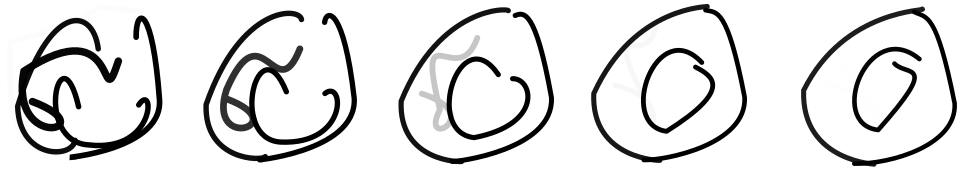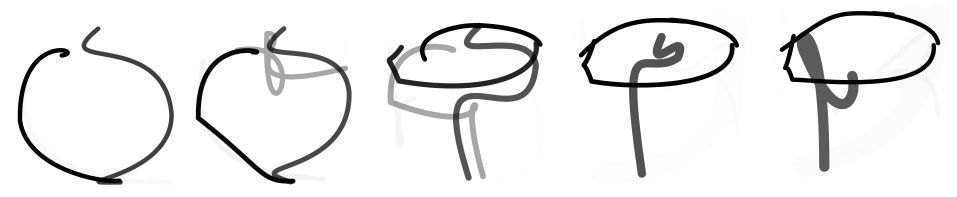Latent space interpolation examples.
-->